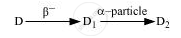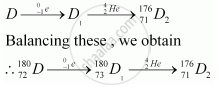Advertisement Remove all ads

# The Radioactive Isotope D Decays According to the Sequenceif the Mass Number and Atomic Number of D2 Are 176 and 71 Respectively, What is (I) the Mass Number (Ii) Atomic Number of D? - Physics

Advertisement Remove all ads
Advertisement Remove all ads
Advertisement Remove all ads

The radioactive isotope D decays according to the sequenceIf the mass number and atomic number of D2 are 176 and 71 respectively, what is (i) the mass number (ii) atomic number of D?

Advertisement Remove all ads

#### Solution

An alpha (α) particle is a helium nucleus (""_2^4He)and a beta-minus decay (β) is an emission of an electron.

The substance D2 can be represented as ""_71^176D_2.Hence,

(i) The mass number of D is 180.

(ii) The atomic number of is 72.

Concept: Radioactivity - Law of Radioactive Decay
Is there an error in this question or solution?

#### Video TutorialsVIEW ALL 

Advertisement Remove all ads
Share
Notifications

View all notifications

Forgot password?